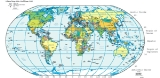xGeographic coordinate systemOverview

A geographic coordinate system is a coordinate system
Coordinate system
In geometry, a coordinate system is a system which uses one or more numbers, or coordinates, to uniquely determine the position of a point or other geometric element. The order of the coordinates is significant and they are sometimes identified by their position in an ordered tuple and sometimes by...

that enables every location on the Earth to be specified by a set of numbers. The coordinates are often chosen such that one of the numbers represent vertical position
Altitude
Altitude or height is defined based on the context in which it is used . As a general definition, altitude is a distance measurement, usually in the vertical or "up" direction, between a reference datum and a point or object. The reference datum also often varies according to the context...

, and two or three
N-vector
n-vector is a three parameter non-singular horizontal position representation well-suited for replacing latitude and longitude in mathematical calculations and computer algorithms. Geometrically, it is a unit vector that is normal to the reference ellipsoid. The vector is decomposed in an Earth...

of the numbers represent horizontal position
Horizontal position representation
A position representation is the parameters used to express a position relative to a reference. Representing position in three dimensions is often done by a Euclidean vector. However, when representing position relative to the Earth it is often more convenient to represent vertical position as...

. A common choice of coordinates is latitude
Latitude
In geography, the latitude of a location on the Earth is the angular distance of that location south or north of the Equator. The latitude is an angle, and is usually measured in degrees . The equator has a latitude of 0°, the North pole has a latitude of 90° north , and the South pole has a...

, longitude
Longitude
Longitude is a geographic coordinate that specifies the east-west position of a point on the Earth's surface. It is an angular measurement, usually expressed in degrees, minutes and seconds, and denoted by the Greek letter lambda ....

and elevation
Elevation
The elevation of a geographic location is its height above a fixed reference point, most commonly a reference geoid, a mathematical model of the Earth's sea level as an equipotential gravitational surface ....

.
The geographic latitude
Latitude
In geography, the latitude of a location on the Earth is the angular distance of that location south or north of the Equator. The latitude is an angle, and is usually measured in degrees . The equator has a latitude of 0°, the North pole has a latitude of 90° north , and the South pole has a...

(abbreviation: Lat., φ, or phi) of a point on the Earth's surface is the angle between the equatorial plane and a line that passes through that point and is normal
Surface normal
A surface normal, or simply normal, to a flat surface is a vector that is perpendicular to that surface. A normal to a non-flat surface at a point P on the surface is a vector perpendicular to the tangent plane to that surface at P. The word "normal" is also used as an adjective: a line normal to a...

to the surface of a reference ellipsoid
Reference ellipsoid
In geodesy, a reference ellipsoid is a mathematically-defined surface that approximates the geoid, the truer figure of the Earth, or other planetary body....

which approximates the shape of the Earth
Figure of the Earth
The expression figure of the Earth has various meanings in geodesy according to the way it is used and the precision with which the Earth's size and shape is to be defined. The actual topographic surface is most apparent with its variety of land forms and water areas. This is, in fact, the surface...

.The surface of the Earth is closer to an ellipsoid
Earth ellipsoid
An Earth ellipsoid is a mathematical figure approximating the shape of the Earth, used as a reference frame for computations in geodesy, astronomy and the geosciences...

than to a sphere, as its equatorial diameter is larger than its north-south diameter.
This line passes a few kilometers away from the center of the Earth except at the poles and the equator where it passes through Earth's center.The greatest distance between an ellipsoid normal and the center of the Earth is 21.9 km at a latitude of 45°, using Earth radius#Radius at a given geodetic latitude and Latitude#Comparison of selected types: Lines joining points of the same latitude trace circles on the surface of the Earth called parallels
Circle of latitude
A circle of latitude, on the Earth, is an imaginary east-west circle connecting all locations that share a given latitude...

, as they are parallel to the equator and to each other.